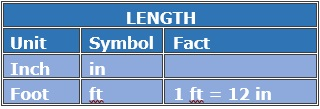# Adding Measurements in Feet and Inches

#### Complete Python Prime Pack

9 Courses     2 eBooks

#### Artificial Intelligence & Machine Learning Prime Pack

6 Courses     1 eBooks

#### Java Prime Pack

9 Courses     2 eBooksAdd 5 feet 2 in + 7 ft 11 in

### Solution

Step 1:

1 ft = 12 in

Step 2:

Converting feet into inches and adding

5 ft 2 in + 7 ft 11 in = [5 x 12 + 2] + [7 x 12 + 11] = 62 + 95 = 157 in

Step 3:

Converting inches into feet

157 in = $\frac{157}{12}$ ft = 13 ft 1 in

Step 4:

So, 5 feet 2 in + 7 ft 11 in = 13 ft 1 in

Add 8 feet 5 in + 12 ft 9 in

### Solution

Step 1:

1 ft = 12 in

Step 2:

Converting feet into inches and adding

8 ft 5 in + 12 ft 9 in = [8 x 12 + 5] + [12 x 12 + 9] = 101 + 153 = 254 in

Step 3:

Converting inches into feet

254 in = $\frac{254}{12}$ ft = 21 ft 2 in

Step 4:

So, 8 feet 5 in + 12 ft 9 in = 21 ft 2 in